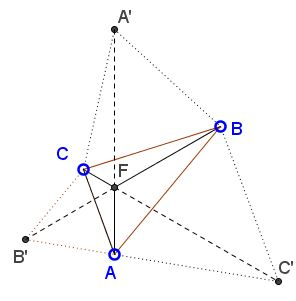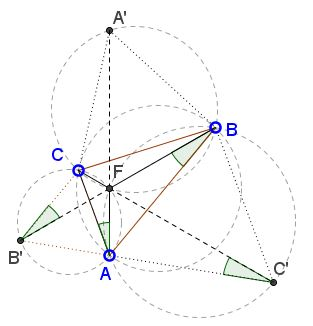# Fermat in a Triangle with a 60 Degrees Angle

### Problem

Let $ABC\;$ be a triangle with $\angle A=60^{\circ}.\;$ Let $F\;$ be the First Fermat Point.Then $AF^2=BF\cdot CF..$

### Proof 1

Since $\angle A=60^{\circ},\;$ all of the angles are less than $120^{\circ},\;$ so that $F\;$ is strictly inside the triangle. WLOG, choose $F=0,\;$ $A=1,\;$ $B=bw,\;$ $C=sw^2,\;$ where $w=\displaystyle -\frac{1}{2}+i\frac{\sqrt{3}}{2}.\;$ Then, $\displaystyle\frac{C-A}{B-A}=\frac{AC}{BC}\cdot \left(\cos\frac{\pi}{3}+i\sin\frac{\pi}{3}\right).\;$ It follows that $\displaystyle\frac{\Im\left(\frac{cw^2-1}{bw-1}\right)}{\Re\left(\frac{cw^2-1}{bw-1}\right)}=\sqrt{3}.\;$ On the other hand, $\displaystyle\frac{cw^2-1}{bw-1}=\frac{(cw^2-1)(bw^2-1)}{|bw-1|^2}\;$ so that

\displaystyle\begin{align} (cw^2-1)(bw^2-1)&=bcw-(b+c)w^2+1\\ &=bc\left(-\frac{1}{2}+i\frac{\sqrt{3}}{2}\right)+(b+c)bc\left(\frac{1}{2}+i\frac{\sqrt{3}}{2}\right)+1\\ &=\frac{1}{2}[(b_c-bc+2)+i(b+c+bc)\sqrt{3}]. \end{align}

From here, $\displaystyle\frac{(b+c+bc)\sqrt{3}}{b+c-bc+2}=\sqrt{3},$ i.e., $b+c+bc=b+c-bc+2,\;$ or $bc=1.\;$

### Proof 2

$F\;$ is known to be the intersection of lines $AA',\;$ $BB',\;$ $CC'\;$ where $CBA',\;$ $ACB',\;$ and $BAC'\;$ are external Napoleon triangles. In addition, the three segments meet at $120^{\circ}\;$ angles.We'll chase angles. In $\Delta B'C'F,\;$ $\angle B'FC'=120^{\circ}\;$ so that $\angle AB'F+\angle AC'F=60^{\circ}.\;$ In $\Delta ACB',\;$ $\angle AB'F+\angle FB'C=60^{\circ},\;$ making $\angle AC'F=\angle FB'C.\;$ Further, $\angle AC'F=\angle ABF,\;$ as inscribed in $(ABC'),\;$ whereas $\angle FB'C=\angle FAC,\;$ as inscribed in $(ACB').$ It follows that $\angle FAC=\angle FBA.\;$ In addition, $\angle AFB=\angle AFC=120^{\circ}.\;$ Thus triangles $ABF\;$ and $CAF\;$ are similar: $\displaystyle\frac{AF}{AC}=\frac{BF}{AF}.$

### Acknowledgment

The problem has been posted by Leo Giugiuc at the CutTheKnotMath facebook page. The problem and Proof 1 are due to Ruben Dario,Dan Sitaru and Leo Giugiuc.